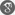##### Cite as:
Mohamed Zellal, Kacem Belghaba, He’s Variational Iteration Method for Solving Multi-Dimensional of Navier Stokes Equation, Int. J. Anal. Appl., 18 (5) (2020), 724-737.

#### Abstract

In this paper, He’s variational iteration method (VIM), established by He in (1999), is adopted to solve two and three dimensional of Navier-Stokes equation in cartesian coordinates. This method is a powerful tool to handle linear and nonlinear models. The main property of the method is its softness and ability to solve nonlinear equations, accurately and easily. Using variational iteration method, it is possible to find the exact solution or a closed approximate solution of a problem. To illustrate the capacity and reliability of this method, some examples and numerical results are provided.

#### References

1. M.A. Abdou, A.A. Soliman, Variational iteration method for solving Burger’s and coupled Burger’s equations, J. Comput. Appl. Math. 181 (2005), 245–251.2. A. AlSaif, Analytical approximate solutions for two-dimensional incompressible Navier-Stokes equations, Adv. Phys. Theor. Appl. 49 (2015), 69–86.3. B. Batiha, M.S.M. Noorani, I. Hashim, Variational iteration method for solving multispecies Lotka–Volterra equations, Comput. Math. Appl. 54 (2007), 903–909.4. J. Biazar, H. Aminikhah, Exact and numerical solutions for non-linear Burger’s equation by VIM, Math. Computer Model. 49 (2009), 1394–1400.5. B.K. Singh, P. Kumar, FRDTM for numerical simulation of multi-dimensional, time-fractional model of Navier–Stokes equation, Ain Shams Eng. J. 9 (2018), 827–834.6. M. Dehghan, F. Shakeri, Application of He’s variational iteration method for solving the Cauchy reaction–diffusion problem, J. Comput. Appl. Math. 214 (2008), 435–446.7. M. Dehghan, M. Tatari, The use of He’s variational iteration method for solving the Fokker-Planck equation, Phys. Scr. 74 (2006), 310–316.8. M. El-Shahed, A. Salem, On the generalized Navier–Stokes equations, Appl. Math. Comput. 156 (2005), 287–293.9. J.H. He, Variational iteration method for delay differential equations, Commun. Nonlinear Sci. Numer. Simul. 2 (4) (1997), 235–236.10. J.H. He, Variational iteration method–A kind of non-linear analytical technique: Some examples, Internat. J. Non-Linear Mech. 34 (1999), 699–708.11. J.H. He, Variational iteration method for autonomous ordinary differential systems, Appl. Math. Comput. 114 (2/3) (2000), 115–123.12. J.H. He, X.H. Wu, Construction of solitary solution and compacton-like solution by variational iteration method, Chaos Solitons Fractals. 29 (2006), 108–113.13. J. H. He, Variational iteration method-Some recent results and new interpretations, J. Comput. Appl. Math. 207 (2007), 3–17.14. J. H. He,, X. H. Wu, Variational iteration method: New development and applications, Comput. Math. Appl. 54 (7-8) (2007), 881–894.15. S. Kumar, D. Kumar, S. Abbasbandy, and M. M. Rashidi, Analytical Solution of Fractional Navier-Stokes Equation by using Modified Laplace Decomposition Method, Ain Shams Eng. J. 5 (2) (2014), 569–574.16. S. Momani, S. Abuasad, Application of He’s variational iteration method to Helmholtz equation, Choas Solitons Fractals. 27 (2006), 1119–1123.17. S. Momani, Z. Odibat, Analytical solution of a time-fractional Navier–Stokes equation by Adomian decomposition method, Appl. Math. Comput. 177 (2) (2006), 488–494.18. Z. M. Odibat, A study on the convergence of variational iteration method, Math. Computer Model. 51 (2010), 1181–1192.19. X. Shang, D. Han, Application of the variational iteration method for solving nth-order integro-differential equations, J. Comput. Appl. Math. 234 (2010), 1442–1447.20. M. Tatari, M. Dehghan, He’s variational iteration method for computing a control parameter in a semi-linear inverse parabolic equation, Chaos Solitons Fractals. 33 (2007), 671–677.21. M. Tatari, M. Dehghan, On the convergence of He’s variational iteration method, J. Comput. Appl. Math. 207 (2007), 121–128.22. N. Thamareerat, A. Luadsong, N. Aschariyaphotha, The meshless local Petrov–Galerkin method based on moving Kriging interpolation for solving the time fractional Navier–Stokes equations, SpringerPlus. 5 (2016), 417.23. H. A. Wahab, J. Anwar, B. Saira, N. Muhammad, S. Muhammad and H. Sajjad, Application of homotopy perturbation method to the Navier-Stokes equations in cylindrical coordinates, Comput. Ecol. Softw. 5 (2) (2015), 139–151.24. A. M. Wazwaz, The variational iteration method: A powerful scheme for handling linear and nonlinear diffusion equations, Comput. Math. Appl. 54 (2007), 933–939.25. A. M. Wazwaz, The variational iteration method for solving linear and nonlinear systems of PDEs, Comput. Math. Appl. 54 (2007), 895–902.26. A. M. Wazwaz, The variational iteration method for rational solutions for KdV, K(2,2), Burgers, and cubic Boussinesq equations, J. Comput. Appl. Math. 207 (2007), 18–23.27. A. M. Wazwaz, The variational iteration method for analytic treatment for linear and nonlinear ODEs, Appl. Math. Comput. 212 (2009), 120–134.28. M. Zellal, K. Belghaba, An accurate algorithm for solving biological population model by the variational iteration method using He’s polynomials, Arab J. Basic Appl. Sci. 25 (3) (2018), 142–149.